# Chemistry Topic-Wise Test - 10

## 45 Questions MCQ Test NEET Mock Test Series & Past Year Papers | Chemistry Topic-Wise Test - 10

Description
This mock test of Chemistry Topic-Wise Test - 10 for NEET helps you for every NEET entrance exam. This contains 45 Multiple Choice Questions for NEET Chemistry Topic-Wise Test - 10 (mcq) to study with solutions a complete question bank. The solved questions answers in this Chemistry Topic-Wise Test - 10 quiz give you a good mix of easy questions and tough questions. NEET students definitely take this Chemistry Topic-Wise Test - 10 exercise for a better result in the exam. You can find other Chemistry Topic-Wise Test - 10 extra questions, long questions & short questions for NEET on EduRev as well by searching above.
QUESTION: 1

Solution:
QUESTION: 2

Solution:
QUESTION: 3

### Concentrated nitric acid is a

Solution:
QUESTION: 4

Which of the following metals is rendered passive by the action of concentrated
HNO3

Solution:
QUESTION: 5
Which of the following strucutres represents pyrophosphoric acid ?
Solution:
QUESTION: 6
Which of the following acids is used in lead storage batteries as an electrolyte ?
Solution:
QUESTION: 7
Which of the following bonds has the highest energy ?
Solution:
QUESTION: 8
The colour of bromine (liquid) is
Solution:
QUESTION: 9

Chlorine reacts with dilute NaOH under ordinary conditions to give

Solution:
QUESTION: 10
Conectrated HCl contains
Solution:
QUESTION: 11

XeF4 reacts violently with water to give

Solution:
QUESTION: 12

Which of the following compounds on heating at about 140 °C gives acetic acid ?

Solution:
QUESTION: 13

Trichloromethane on heating with aqueous KOH and subsequent treatment with dilute HCl gives

Solution:
QUESTION: 14

Consider the following sequence of reactions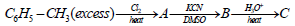The product (C) is

Solution:
QUESTION: 15
The compound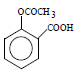Solution:
QUESTION: 16

The following reactions are carried out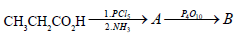The end product (B) is

Solution:
QUESTION: 17

Which of the following compounds will not liberate ammonia gas on refluxing with aqueous NaOH ?

Solution:
QUESTION: 18
The major product formed in the reaction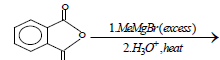is
Solution:
QUESTION: 19

Which of the following is a chiral molecule ?

Solution:

The structure of 2-methylbutanal is CH3-CH2-CH(CH3)-CHO. The 2nd carbon atom (counting from the aldehyde group) is attached to different groups. so it is a chiral molecule.

QUESTION: 20

Consider the following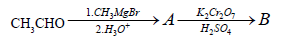sequence of reactions
The product (B) is

Solution:
QUESTION: 21

The reagent that can distinguish benzaldehyde from propionaldehyde is

Solution:
QUESTION: 22

Which of the following will react with water ?

Solution:
QUESTION: 23

A secondary amine is a compound which possess

Solution:
QUESTION: 24
Consider the following reactions of the compound (X)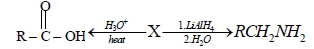The compound (X) is expected to be
Solution:
QUESTION: 25
Consider the following compounds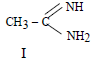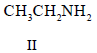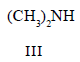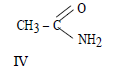The correct order of basicity of the above compounds is
Solution:
QUESTION: 26
Among the following, the strongest base is
Solution:
QUESTION: 27
Which of the following carbohydrates is a monosaccharide
Solution:
QUESTION: 28
Solution:
QUESTION: 29
Mutarotation involves
Solution:
QUESTION: 30

The smallest chiral α - amino acid is

Solution:
QUESTION: 31
Which of the following polyamides is a polypeptide ?
Solution:
QUESTION: 32
Which of the following enzymes is secreted by the pancreas into the small intenstine ?
Solution:
QUESTION: 33
Which of the following is commonly used as an intravenous general anaesthetic ?
Solution:
QUESTION: 34
The bright red dye that became the first sulphonamide drug to treat bacterial infection as discovered by Domagk is
Solution:
QUESTION: 35
The instantaneous rate of reaction is given by the expression
Solution:
QUESTION: 36

For a zero-order reaction of the type A → products, the rate equation may be expressed as

Solution:
QUESTION: 37

The units of specific reaction rate for a second-order reaction is

Solution:
QUESTION: 38
The half-life period for a first-order reaction is 10 minutes. How much time is reqired to change the
conentration of the reactant from 0.08 M to 0.01 M ?
Solution:
QUESTION: 39
For an elementary reaction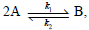the rate of disappearance of A is equal to
Solution:
QUESTION: 40

The density of copper is 8.94 g/L find the charge needed to plate an area of  10 x 10cmto a thickness
of 10-2 to a thickness of CuSo4 solution as electrolyte(At.wt of Cu = 63.6)

Solution:
QUESTION: 41

Calculate the standard free energy change for the reaction 2Ag + 2H+ → H2 + 2Ag+
E0 for Ag+ +e→ Ag is 0.8 V

Solution:
QUESTION: 42
Four alkali metals A,B,C and D are having respectively standard electrode potentials as –3.05, –1.66, –0.40 and 0.80 V. Which one will be most reducing
Solution:
QUESTION: 43
A standard hydrogen electrode has zero electrode potential because
Solution:
QUESTION: 44

Zn gives H2 gas with H2So& HCl but not with HNObecause

Solution:
QUESTION: 45

The correct order of decreasing acid strength of

trichloroacetic acid (A),

trifluoroacetic acid (B),

acetic acid (C)

and formic acid (D) is

Solution: